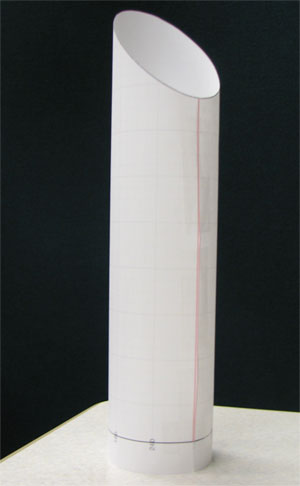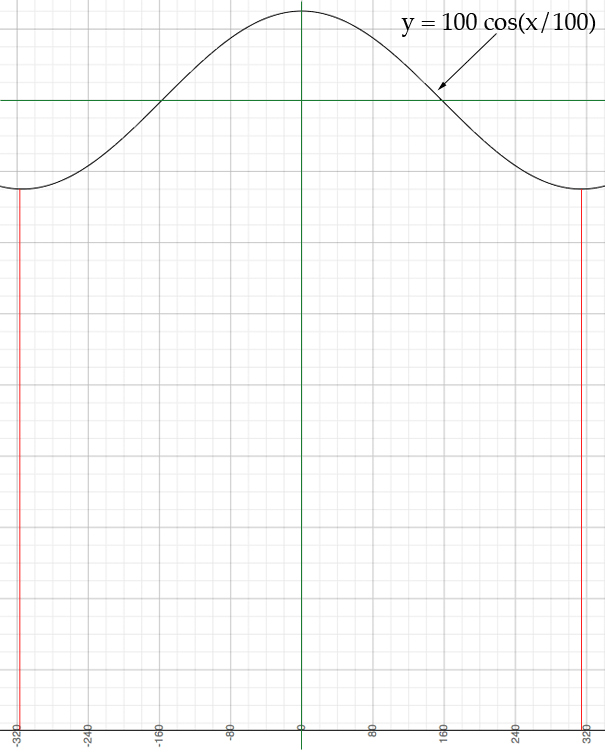SEARCH HOMEMath Central Quandaries & QueriesQuestion from Al: i want to cut a 200 diameter pipe in 45 degrees. Can you demonstrate how to develop a flat rap around pleaseHi Al,We had a similar question a while ago and Edward showed that the curve is a cosine. For your question the curve you need is one period of the cosine. The length of the period is the circumference of the pipe which is 200 π and the amplitude is 200/2 = 100. (You didn't tell us the units but I assume they are millimeters.) Thus the curve is

y = 100 cos(x/100)

where the angle is measured in radians, not degrees. In the diagram the axes are in green. The red lines mark off one period.I printed the diagram, cut along the curve and red lines and rolled it up to form the cut off cylinder in the image at the top of this page.

I hope this helps,
HarleyMath Central is supported by the University of Regina and The Pacific Institute for the Mathematical Sciences.